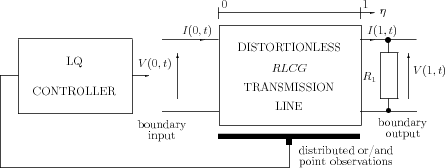]> 8.2 Statement of the lq problem

### 8.2 Statement of the lq problem

Consider a controlled distortionless $RLCG$ transmission line, i.e. $R∕L=G∕C$, loaded by a resistance ${R}_{1}$ and depicted in Figure 8.1.Figure 8.1: The control system under study

The system is governed by the equations

 $\left\{\begin{array}{ccccc}\hfill L\frac{\partial I\left(\eta ,t\right)}{\partial t}& \hfill =\hfill & -\frac{\partial V\left(\eta ,t\right)}{\partial \eta }-RI\left(\eta ,t\right),\hfill & \phantom{\rule{1em}{0ex}}0\le \eta \le 1,\hfill & \phantom{\rule{0ex}{0ex}}t\ge 0\hfill \\ \hfill C\frac{\partial V\left(\eta ,t\right)}{\partial t}& \hfill =\hfill & -\frac{\partial I\left(\eta ,t\right)}{\partial \eta }-GV\left(\eta ,t\right),\hfill & \phantom{\rule{1em}{0ex}}0\le \eta \le 1,\hfill & \phantom{\rule{0ex}{0ex}}t\ge 0\hfill \\ \hfill I\left(1,t\right){R}_{1}& \hfill =\hfill & V\left(1,t\right),\hfill & \hfill & \phantom{\rule{0ex}{0ex}}t\ge 0\hfill \\ \hfill V\left(0,t\right)& \hfill =\hfill & u\left(t\right),\hfill & \hfill & \phantom{\rule{0ex}{0ex}}t\ge 0\hfill \\ \hfill y\left(t\right)& \hfill =\hfill & V\left(1,t\right),\hfill & \hfill & \phantom{\rule{0ex}{0ex}}t\ge 0\hfill \end{array}\right\}$ (8.1)

The control is an input voltage and its goal is to minimize the sum of energy of the input and output signals expressed by the performance index

 $J={\int }_{0}^{\infty }\left[{y}^{2}\left(t\right)+{u}^{2}\left(t\right)\right]dt$ (8.2)

The d’Alembert solutions of the ﬁrst two equations take the form (3.8) with $\alpha =R∕L=G∕C$. Substituting (3.8) into boundary conditions, we get the system of functional equations

 $\left\{\begin{array}{ccc}\hfill \Psi \left(1+\nu t\right)& \hfill =\hfill & \kappa \Phi \left(1-\nu t\right)\hfill \\ \hfill \frac{1}{2}{e}^{-\alpha t}\left[\Phi \left(-\nu t\right)+\Psi \left(\nu t\right)\right]& \hfill =\hfill & u\left(t\right)\hfill \\ \hfill y\left(t\right)& \hfill =\hfill & \frac{1}{2}{e}^{-\alpha t}\left[\Phi \left(1-\nu t\right)+\Psi \left(1+\nu t\right)\right]\hfill \end{array}\right\}$ (8.3)

where $\kappa =\left({R}_{1}-Z\right)∕\left({R}_{1}+Z\right)$ is the reﬂection coefficient. Introducing the new variables

 $\left\{\begin{array}{ccc}\hfill {z}_{1}\left(t\right)& \hfill =\hfill & \frac{1}{2}{e}^{-\alpha t}{e}^{\alpha r}\Phi \left(1-\nu t\right)\hfill \\ \hfill {z}_{2}\left(t\right)& \hfill =\hfill & \frac{1}{2}{e}^{-\alpha t}\Phi \left(-\nu t\right)\hfill \end{array}\right\}$ (8.4)

one obtains

 $\left\{\begin{array}{ccc}\hfill z\left(t\right)& \hfill =\hfill & Az\left(t-r\right)+u\left(t\right){b}_{0}\hfill \\ \hfill y\left(t\right)& \hfill =\hfill & {c}_{0}^{\ast }z\left(t-r\right)\hfill \end{array}\right\}$ (8.5)

here,

$z\left(t\right)=\left[\begin{array}{c}{z}_{1}\left(t\right)\hfill \\ {z}_{2}\left(t\right)\hfill \end{array}\right],\phantom{\rule{1em}{0ex}}A=\left[\begin{array}{cc}\hfill 0& \hfill 1\\ \hfill -b& \hfill 0\end{array}\right],\phantom{\rule{1em}{0ex}}{b}_{0}=\left[\begin{array}{c}\hfill 0\hfill \\ \hfill 1\hfill \end{array}\right],\phantom{\rule{1em}{0ex}}{c}_{0}=\left[\begin{array}{c}\hfill 0\hfill \\ \hfill a\hfill \end{array}\right],$

where $a:=\frac{1+\kappa }{\rho }\ge 0$, $b:=\frac{\kappa }{{\rho }^{2}}$, $\left|b\right|<1$, $\rho :={e}^{\alpha r}$.